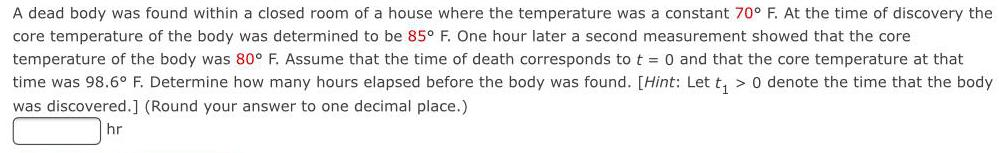Question:

# A dead body was found within a closed room of a house where

Last updated: 7/10/2022A dead body was found within a closed room of a house where the temperature was a constant 70° F. At the time of discovery the core temperature of the body was determined to be 85° F. One hour later a second measurement showed that the core temperature of the body was 80° F. Assume that the time of death corresponds to t = 0 and that the core temperature at that time was 98.6° F. Determine how many hours elapsed before the body was found. [Hint: Let t₁ > 0 denote the time that the body was discovered.] (Round your answer to one decimal place.)